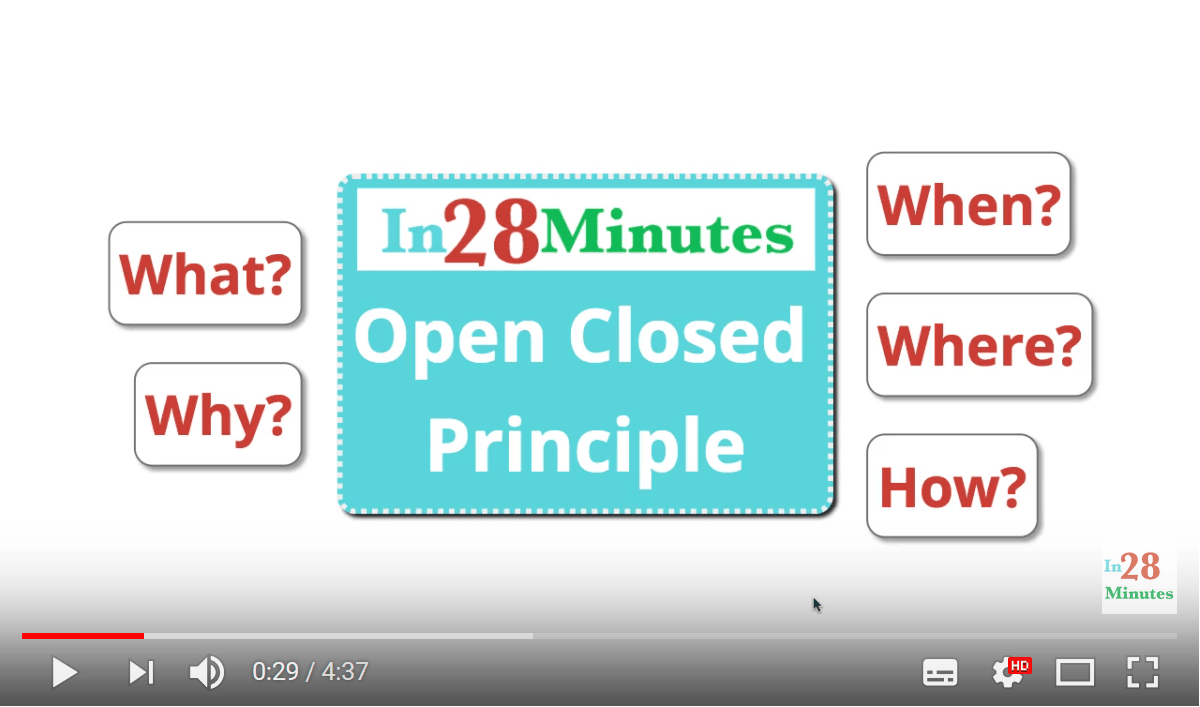Open Closed Principle is one of the SOLID Principles. You want your code to be extensible. How do you achieve it with minimum fuss? Let’s get started.

### We learn

• What is the Open Closed Principle?
• How do you apply this principle in developing and designing software applications?

### Software Design Principles

This is the fifth article in a series of articles on important Software Design Principles:

## Open Closed Principle

Your classes should be open to extension, but closed to modification

What does this mean? Let’s look at a simple example to understand what this means.

### An example of Poor Design

Let’s take a quick look at the calculateArea method in the Shape class.

class Shape {
public double calculateArea(Shape[] shapes) {
double area = 0;
for(Shape shape:shapes) {
if(shape instanceof Rectangle) {
//Calculate Area of Rectange
}
else if(shape instanceof Circle) {
//Calculate Area of Circle
}
}
return area;
}
}

class Rectangle extends Shape {

}

class Circle extends Shape {

}



Is there a problem with calculateArea() method?

What if we add a new shape? What if we remove a shape? What if we want to change the area algorithm for one of the shapes.

For all these modifications, calculateArea() method needs to change.

How can we make it better?

Parts of the above code below pseudo code, for ease of explanation


abstract class Shape {

abstract double area();
}

class Rectangle extends Shape {

@Override
double area() {
// Area implementation for Rectangle
return 0;
}

}

class Circle extends Shape {

@Override
double area() {
// Area implementation for Rectangle
return 0;
}

}



A better solution would be to allow each of the shapes, to define their own area() method. We have created an abstract class called Shape (which could also have been an interface), and have each of the different shapes extend it. Each shape also overrides Shape’s abstract area() method, to compute its specific area.

The standalone calculateArea() method would now look like this:

abstract class Shape {

public double calculateArea(Shape[] shapes)
{
double area = 0;
for(Shape shape:shapes) {
area += shape.area();
}
return area;
}

abstract double area();
}



calculateArea() is now responsible just for looping around the shapes, and invoking the area() method of individual shapes.

This is a very good example of the OCP.

If you now want to add another shape, then you need to extend the Shape class, and override its area() method:. That’s it.

Here, Shape class is open to extension, and calculateArea() is closed to modification.

Do check out our video on this: